# Moran’s Eigenvector Maps and related methods for the spatial multiscale analysis of ecological data

#### 2019-06-04

The package adespatial contains functions for the multiscale analysis of spatial multivariate data. It implements some new functions and reimplements existing functions that were available in packages of the sedaR project hosted on R-Forge (spacemakeR, packfor, AEM, etc.). It can be seen as a bridge between packages dealing with mutltivariate data (e.g., ade4, Dray and Dufour (2007)) and packages that deals with spatial data (sp, spdep). In adespatial, many methods consider the spatial information as a spatial weighting matrix (SWM), object of class listw provided by the spdep package (Figure 1). The SWM is defined as the Hadamard product (element-wise product) of a connectivity matrix by a weighting matrix. The binary connectivity matrix (spatial neighborhood, object of class nb) defines the pairs of connected and unconnected samples, while the weighting matrix allows weighting the connections, for instance to define that the strength of the connection between two samples decreases with the geographic distance.

Once SWM is defined, it can be used to build Moran’s Eigenvector Maps (MEM, Dray, Legendre, and Peres-Neto (2006)) that are orthogonal vectors maximizing the spatial autocorrelation (measured by Moran’s coefficient). These spatial predictors can be used in multivariate statistical methods to provide spatially-explicit multiscale tools (Dray et al. 2012). This document provides a description of the main functionalities of the package.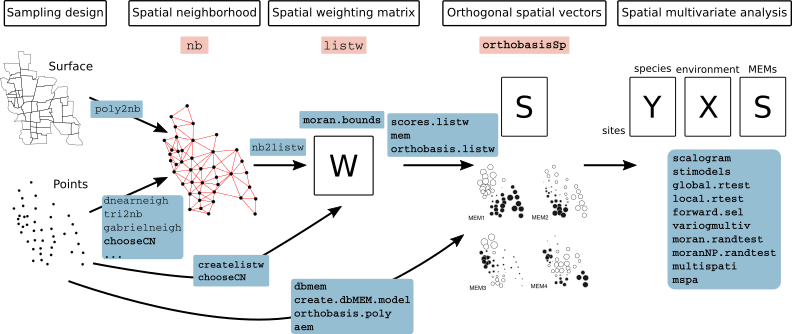Figure 1: Schematic representation of the functioning of the adespatial package. Classes are represented in pink frames and functions in blue frames. Classes and functions provided by adespatial are in bold.

To run the different analysis described, several packages are required and are loaded:

library(adespatial)
library(ade4)
##
## Attaching package: 'ade4'
## The following object is masked from 'package:adespatial':
##
##     multispati
library(adegraphics)
##
## Attaching package: 'adegraphics'
## The following objects are masked from 'package:ade4':
##
##     kplotsepan.coa, s.arrow, s.class, s.corcircle, s.distri,
##     s.image, s.label, s.logo, s.match, s.traject, s.value,
##     table.value, triangle.class
library(spdep)
## Loading required package: sp
## Loading required package: spData
## To access larger datasets in this package, install the spDataLarge
## package with: install.packages('spDataLarge',
## repos='https://nowosad.github.io/drat/', type='source')
## Loading required package: sf
## Linking to GEOS 3.6.2, GDAL 2.2.3, PROJ 4.9.3
##
## Attaching package: 'spdep'
## The following object is masked from 'package:ade4':
##
##     mstree
library(maptools)
## Checking rgeos availability: TRUE

# 1 Building spatial neighborhood

Spatial neighborhoods are managed in spdep as objects of class nb. It corresponds to the notion of connectivity matrices discussed in Dray, Legendre, and Peres-Neto (2006) and can be represented by an unweighted graph. Various functions allow to create nb objects from geographic coordinates of sites. We present different alternatives according to the design of the sampling scheme.

## 1.1 Surface data

The function poly2nb allows to define neighborhood when the sampling sites are polygons and not points (two regions are neighbors if they share a common boundary). The resulting object can be plotted on a geographical map using the s.Spatial function of the adegraphics package (Siberchicot et al. 2017).

data(mafragh)
class(mafragh$Spatial) ##  "SpatialPolygons" ## attr(,"package") ##  "sp" nb.maf <- poly2nb(mafragh$Spatial)
##  7
##
## $comp.id ##  1 2 3 3 2 4 5 5 6 4 6 6 2 6 7 7 5 2 7 1 More elaborate procedures are available to define neighborhood. For instance, Delaunay triangulation is obtained with the function tri2nb. It requires the package deldir. Other graph-based procedures are also available: nbtri <- tri2nb(xyir) ## ## PLEASE NOTE: The components "delsgs" and "summary" of the ## object returned by deldir() are now DATA FRAMES rather than ## matrices (as they were prior to release 0.0-18). ## See help("deldir"). ## ## PLEASE NOTE: The process that deldir() uses for determining ## duplicated points has changed from that used in version ## 0.0-9 of this package (and previously). See help("deldir"). nbgab <- graph2nb(gabrielneigh(xyir), sym = TRUE) nbrel <- graph2nb(relativeneigh(xyir), sym = TRUE) nbsoi <- graph2nb(soi.graph(nbtri, xyir), sym = TRUE) g1 <- s.label(xyir, nb = nbtri, pnb.edge.col = "red", main = "Delaunay", plot = FALSE) g2 <- s.label(xyir, nb = nbgab, pnb.edge.col = "red", main = "Gabriel", plot = FALSE) g3 <- s.label(xyir, nb = nbrel, pnb.edge.col = "red", main = "Relative", plot = FALSE) g4 <- s.label(xyir, nb = nbsoi, pnb.edge.col = "red", main = "Sphere of influence", plot = FALSE) ADEgS(list(g1, g2, g3, g4))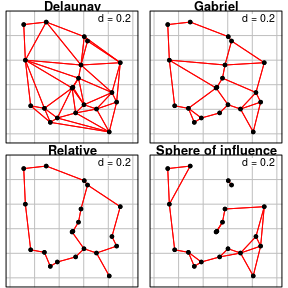The adespatial functions chooseCN and listw.candidates provides simple ways to build spatial neighborhoods. They are wrappers of many of the spdep functions presented above. The function listw.explore is an interactive graphical interface that allows to generate R code to build neighborhood objects (see Figure 2). ## 1.4 Manipulating nb objects A nb object is not stored as a matrix. It is a list of neighbors. The neighbors of the first site are in the first element of the list: nbgab[] ##  7 20 Various tools are provided by spdep to deal with these objects. For instance, it is possible to identify differences between two neighborhoods: diffnb(nbsoi, nbrel) ## Neighbour list object: ## Number of regions: 20 ## Number of nonzero links: 24 ## Percentage nonzero weights: 6 ## Average number of links: 1.2 ## 4 regions with no links: ## 1 4 11 17 Usually, it can be useful to remove some connections due to edge effects. In this case, the function edit.nb provides an interactive tool to add or delete connections. The function include.self allows to include a site in its own list of neighbors (self-loops). The spdep package provides many other tools to manipulate nb objects: intersect.nb(nb.obj1, nb.obj2) union.nb(nb.obj1, nb.obj2) setdiff.nb(nb.obj1, nb.obj2) complement.nb(nb.obj) droplinks(nb, drop, sym = TRUE) nblag(neighbours, maxlag) # 2 Defining spatial weighting matrices A spatial weighting matrices (SWM) is computed by a transformation of a spatial neighborhood. In R, they are not stored as matrices but as objects of the class listw. This format is more efficient than a matrix representation to manage large data sets. An object of class listw can be easily created from an object of class nb with the function nb2listw. Different objects listw can be obtained from a nb object. The argument style allows to define a transformation of the matrix such as standardization by row sum, by total sum or binary coding, etc. General spatial weights can be introduced by the argument glist. This allows to introduce, for instance, a weighting relative to the distances between the points. For this task, the function nbdists is very useful as it computes Euclidean distance between neighbor sites defined by an nb object. To obtain a simple row-standardization, the function is simply called by: nb2listw(nbgab) ## Characteristics of weights list object: ## Neighbour list object: ## Number of regions: 20 ## Number of nonzero links: 58 ## Percentage nonzero weights: 14.5 ## Average number of links: 2.9 ## ## Weights style: W ## Weights constants summary: ## n nn S0 S1 S2 ## W 20 400 20 14.38889 82.06944 More sophisticated forms of spatial weighting matrices can be defined. For instance, it is possible to weight edges between neighbors as functions of geographic distances. In a fist step, distances between neighbors are obtained by the function : distgab <- nbdists(nbgab, xyir) nbgab[] ##  7 20 distgab[] ##  0.3740569 0.1135694 Then, spatial weights are defined as a function of distance (e.g. $$1-d_{ij}/max(d_{ij})$$): fdist <- lapply(distgab, function(x) 1 - x/max(dist(xyir))) And the spatial weighting matrix is then created: listwgab <- nb2listw(nbgab, glist = fdist, style = "B") listwgab ## Characteristics of weights list object: ## Neighbour list object: ## Number of regions: 20 ## Number of nonzero links: 58 ## Percentage nonzero weights: 14.5 ## Average number of links: 2.9 ## ## Weights style: B ## Weights constants summary: ## n nn S0 S1 S2 ## B 20 400 47.49881 79.02568 484.6624 names(listwgab) ##  "style" "neighbours" "weights" listwgab$neighbours[]
##   7 20
listwgab$weights[] ##  0.6652764 0.8983728 The matrix representation of a listw object can also be obtained: print(listw2mat(listwgab)[1:10, 1:10], digits = 3) ## [,1] [,2] [,3] [,4] [,5] [,6] [,7] [,8] [,9] [,10] ## 1 0.000 0 0.00 0.00 0 0.000 0.665 0.000 0.000 0.000 ## 2 0.000 0 0.00 0.00 0 0.000 0.000 0.000 0.000 0.000 ## 3 0.000 0 0.00 0.94 0 0.000 0.000 0.000 0.000 0.000 ## 4 0.000 0 0.94 0.00 0 0.000 0.000 0.000 0.000 0.000 ## 5 0.000 0 0.00 0.00 0 0.000 0.000 0.000 0.000 0.000 ## 6 0.000 0 0.00 0.00 0 0.000 0.000 0.703 0.000 0.960 ## 7 0.665 0 0.00 0.00 0 0.000 0.000 0.000 0.593 0.000 ## 8 0.000 0 0.00 0.00 0 0.703 0.000 0.000 0.000 0.000 ## 9 0.000 0 0.00 0.00 0 0.000 0.593 0.000 0.000 0.819 ## 10 0.000 0 0.00 0.00 0 0.960 0.000 0.000 0.819 0.000 To facilitate the building of spatial neighborhoods (nb object) and associated spatial weighting matrices (listw object), the package adespatial provides several tools. An interactive graphical interface is launched by the call listw.explore() assuming that spatial coordinates are still stored in an object of the R session (Figure 2).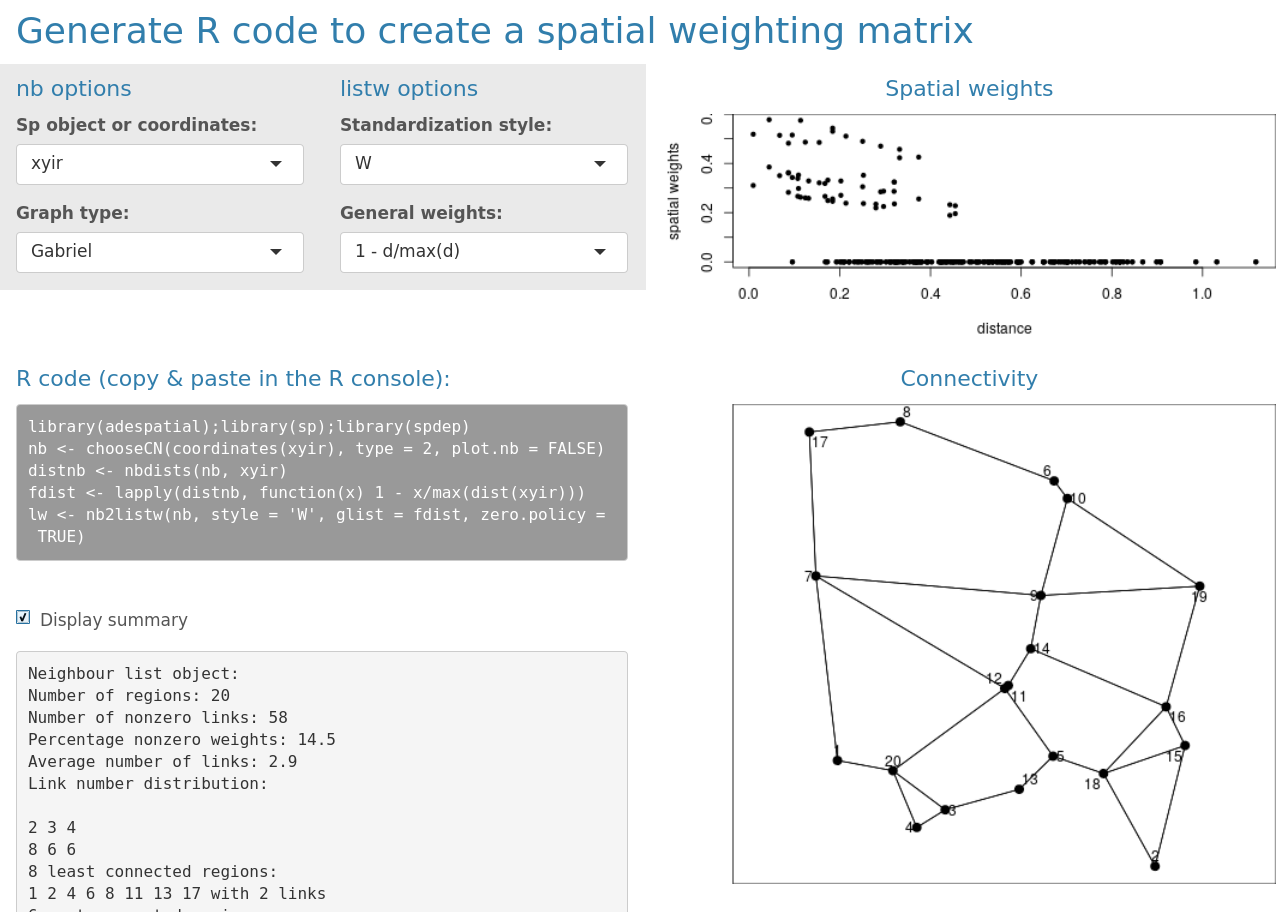Figure 2: The interactive interface provided by the function listw.explore. # 3 Creating spatial predictors The package adespatial provide different tools to build spatial predictors that can be incorporated in multivariate analysis. They are orthogonal vectors stored in a object of class orthobasisSp. Orthogonal polynomials of geographic coordinates can be computed by the function orthobasis.poly whereas principal coordinates of neighbour matrices (PCNM, Borcard and Legendre (2002)) are obtained by the function dbmem. The Moran’s Eigenvectors Maps (MEMs) provide the most flexible framework. If we consider the $$n \times n$$ spatial weighting matrix $$\mathbf{W} = [w_{ij}]$$, they are the $$n-1$$ eigenvectors obtained by the diagonalization of the doubly-centred SWM: $\mathbf{\Omega V}= \mathbf{V \Lambda}$ where $$\mathbf{\Omega} = \mathbf{HWH}$$ is the doubly-centred SWM and $$\mathbf{H} = \left ( \mathbf{I}-\mathbf{11}\hspace{-0.05cm}^{\top}\hspace{-0.05cm}/n \right )$$ is the centring operator. MEMs are orthogonal vectors with a unit norm that maximize Moran’s coefficient of spatial autocorrelation (Griffith 1996; Dray et al. 2012) and are stored in matrix $$\mathbf{V}$$. MEMs are provided by the functions scores.listw or mem of the adespatial package. These two functions are exactly identical (both are kept for historical reasons and compatibility) and return an object of class orthobasisSp. mem.gab <- mem(listwgab) mem.gab ## Orthobasis with 20 rows and 19 columns ## Only 6 rows and 4 columns are shown ## MEM1 MEM2 MEM3 MEM4 ## 1 -0.90672748 -0.06565448 -0.04776682 -0.2371804 ## 2 1.00027097 1.13232055 0.44830373 -0.4506747 ## 3 -1.60466714 0.77623659 1.18177121 1.0279127 ## 4 -1.43085124 0.54646370 1.10065325 1.0434462 ## 5 -0.03992758 1.16673599 -0.92313755 -0.7243739 ## 6 0.15116014 -1.13831331 1.18996867 -0.9394357 This object contains MEMs, stored as a data.frame and other attributes: str(mem.gab) ## Classes 'orthobasisSp', 'orthobasis' and 'data.frame': 20 obs. of 19 variables: ##$ MEM1 : num  -0.9067 1.0003 -1.6047 -1.4309 -0.0399 ...
##  $MEM2 : num -0.0657 1.1323 0.7762 0.5465 1.1667 ... ##$ MEM3 : num  -0.0478 0.4483 1.1818 1.1007 -0.9231 ...
##  $MEM4 : num -0.237 -0.451 1.028 1.043 -0.724 ... ##$ MEM5 : num  -1.61 -0.597 0.434 -0.479 2.175 ...
##  $MEM6 : num 1.48899 0.00604 -0.58245 -0.80351 1.09241 ... ##$ MEM7 : num  0.6065 0.7788 -1.0025 -0.0579 -0.0817 ...
##  $MEM8 : num -0.329 2.48 0.499 0.188 -0.563 ... ##$ MEM9 : num  2.687 -0.631 -0.268 -1.389 0.127 ...
##  $MEM10: num -0.254 -0.824 -0.854 1.464 1.617 ... ##$ MEM11: num  -0.991 -1.722 0.604 0.664 -0.344 ...
##  $MEM12: num -0.864 -0.203 0.171 -1.297 -0.142 ... ##$ MEM13: num  0.0305 -1.0147 0.0403 -1.0781 0.1025 ...
##  $MEM14: num -0.944 0.95 1.627 -2.137 -0.691 ... ##$ MEM15: num  0.403 -1.46 1.732 -0.836 -0.67 ...
##  $MEM16: num -0.101 -0.598 0.366 -0.497 0.655 ... ##$ MEM17: num  -0.863 -0.334 -2.095 0.396 -1.697 ...
##  $MEM18: num -1.215 -0.418 -0.465 -0.397 0.311 ... ##$ MEM19: num  -0.727 0.637 0.021 -0.502 1.703 ...
##  - attr(*, "values")= num  0.1133 0.1005 0.0723 0.0671 0.0551 ...
##  - attr(*, "weights")= num  0.05 0.05 0.05 0.05 0.05 0.05 0.05 0.05 0.05 0.05 ...
##  - attr(*, "call")= language mem(listw = listwgab)

The eigenvalues associated to MEMs are stored in the attribute called values:

    barplot(attr(mem.gab, "values"),
main = "Eigenvalues of the spatial weighting matrix", cex.main = 0.7)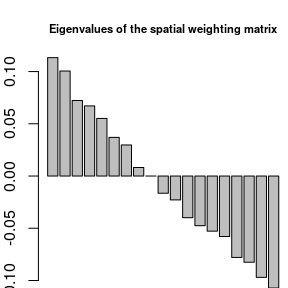A plot method is provided to represent MEMs. By default, eigenvectors are represented as a table (sites as rows, MEMs as columns):

plot(mem.gab)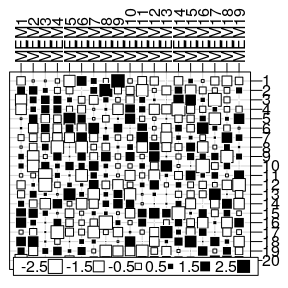The previous representation is not really informative and MEMs can be represented in the geographical space as maps if the argument SpORcoords is documented:

plot(mem.gab, SpORcoords = xyir, nb = nbgab)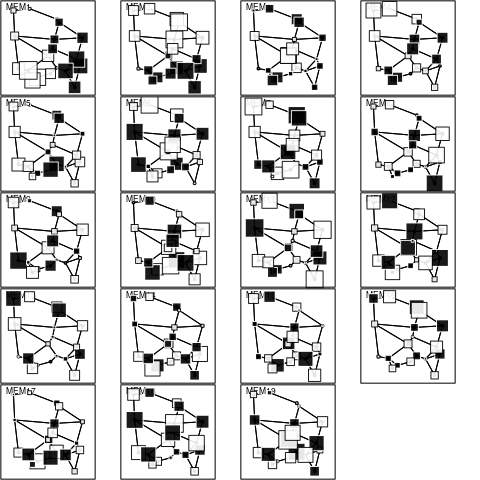Moran’s I can be computed and tested for each eigenvector with the moran.randtest function:

moranI <- moran.randtest(mem.gab, listwgab, 99)
moranI
## class: krandtest lightkrandtest
## Monte-Carlo tests
## Call: moran.randtest(x = mem.gab, listw = listwgab, nrepet = 99)
##
## Number of tests:   19
##
## Adjustment method for multiple comparisons:   none
## Permutation number:   99
##     Test           Obs    Std.Obs   Alter Pvalue
## 1   MEM1  9.545478e-01  5.4108146 greater   0.01
## 2   MEM2  8.463497e-01  5.6654531 greater   0.01
## 3   MEM3  6.087820e-01  3.6902332 greater   0.01
## 4   MEM4  5.651718e-01  3.4503635 greater   0.01
## 5   MEM5  4.642564e-01  3.4428901 greater   0.01
## 6   MEM6  3.114056e-01  2.3805633 greater   0.03
## 7   MEM7  2.503420e-01  1.6708987 greater   0.08
## 8   MEM8  6.873937e-02  0.5738166 greater   0.25
## 9   MEM9  1.108535e-05  0.2289309 greater   0.40
## 10 MEM10 -1.388302e-01 -0.4663896 greater   0.69
## 11 MEM11 -1.923089e-01 -0.8612371 greater   0.81
## 12 MEM12 -3.357687e-01 -1.6790234 greater   0.97
## 13 MEM13 -4.001950e-01 -2.0684406 greater   0.98
## 14 MEM14 -4.445105e-01 -2.4745258 greater   1.00
## 15 MEM15 -4.873813e-01 -2.4327556 greater   0.99
## 16 MEM16 -6.563053e-01 -3.9997778 greater   1.00
## 17 MEM17 -6.947406e-01 -3.9824080 greater   1.00
## 18 MEM18 -8.170207e-01 -4.7431342 greater   1.00
## 19 MEM19 -9.025446e-01 -4.7662544 greater   1.00

By default, the function moran.randtest tests against the alternative hypothesis of positive autocorrelation (alter = "greater") but this can be modified by setting the argument alter to "less" or "two-sided". The function is not only devoted to MEMs and can be used to compute spatial autocorrelations for all kind of variables (see this section).

As demonstrated in Dray, Legendre, and Peres-Neto (2006), eigenvalues and Moran’s I are equal (post-multiply by a constant):

attr(mem.gab, "values") / moranI$obs ## MEM1.statistic MEM2.statistic MEM3.statistic MEM4.statistic ## 0.118747 0.118747 0.118747 0.118747 ## MEM5.statistic MEM6.statistic MEM7.statistic MEM8.statistic ## 0.118747 0.118747 0.118747 0.118747 ## MEM9.statistic MEM10.statistic MEM11.statistic MEM12.statistic ## 0.118747 0.118747 0.118747 0.118747 ## MEM13.statistic MEM14.statistic MEM15.statistic MEM16.statistic ## 0.118747 0.118747 0.118747 0.118747 ## MEM17.statistic MEM18.statistic MEM19.statistic ## 0.118747 0.118747 0.118747 Then, it is possible to map only positive significant eigenvectors (i.e., MEMs with significant positive spatial autocorrelation): signi <- which(moranI$pvalue < 0.05)
signi
##  1 2 3 4 5 6
plot(mem.gab[,signi], SpORcoords = xyir, nb = nbgab)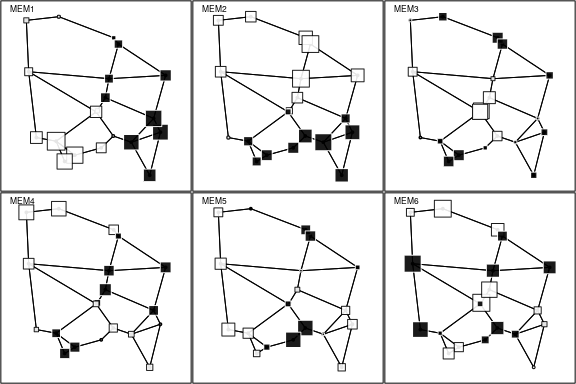# 4 Describing spatial patterns

In the previous sections, we considered only the spatial information as a geographic map, a spatial weighting matrix (SWM) or a basis of spatial predictors (e.g., MEM). In the next sections, we will show how the spatial information can be integrated to analyze multivariate data to identify multiscale spatial patterns. The dataset mafragh available in ade4 will be used to illustrate the different methods.

## 4.1 The Mafragh data set

The Mafragh data set is available in the ade4 package and stored as a list:

data("mafragh")
class(mafragh)
##  "list"
names(mafragh)
##   "xy"              "flo"             "neig"
##   "env"             "partition"       "area"
##   "tre"             "traits"          "nb"
##  "Spatial"         "spenames"        "Spatial.contour"
dim(mafragh$flo) ##  97 56 The data.frame mafragh$flo is a floristic table that contains the abundance of 56 plant species in 97 sites in Algeria. Names of species are listed in mafragh$spenames. The geographic coordinates of the sites are given in mafragh$xy.

str(mafragh$env) ## 'data.frame': 97 obs. of 11 variables: ##$ Clay        : num  0.73 0.75 0.74 0.23 0.73 0.72 0.52 0.42 0.74 0.53 ...
##  $Silt : num 0.24 0.24 0.24 0.26 0.24 0.22 0.46 0.49 0.24 0.44 ... ##$ Sand        : num  0.03 0.02 0.02 0.49 0.03 0.03 0.02 0.08 0.02 0.04 ...
##  $K2O : num 1.3 0.8 1.7 0.3 1.3 1.7 0.8 1.4 1.7 1.4 ... ##$ Mg++        : num  9.2 10.7 8.6 2 9.2 6 6 19.6 8.6 17 ...
##  $Na+/100g : num 4.2 10.4 10.8 1.2 4.2 10.7 18.4 2.5 10.8 11.7 ... ##$ K+          : num  1.2 1.4 1.9 0.3 1.2 1.3 2.2 1.3 1.9 0.5 ...
##  $Conductivity: num 7.9 11.5 10.4 0.6 7.9 14.5 15.2 2.9 10.4 16.9 ... ##$ Retention   : num  41.8 42.4 41.4 22.3 41.8 42.7 37.4 35.4 41.4 38 ...
##  $Na+/l : num 48.7 66 24 2.2 48.7 ... ##$ Elevation   : num  6 2 2 6 6 4 4 3 3 6 ...

The data.frame mafragh$env contains 11 quantitative environmental variables. A map of the study area is also available (mafragh$Spatial) that can be used as a background to display the sampling design:

s.label(mafragh$xy, plabel.cex = 0, ppoint.pch = 15, ppoint.col = "darkseagreen4", Sp = mafragh$Spatial)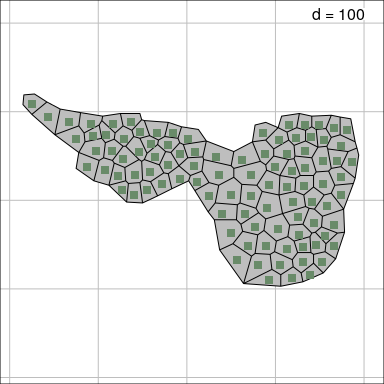A more detailed description of the data set is available at http://pbil.univ-lyon1.fr/R/pdf/pps053.pdf (in French).

The functionalities of the adegraphics package (Siberchicot et al. 2017) can be used to design simple thematic maps to represent data. For instance, it is possible to represent the spatial distribution of two species using the s.Spatial function:

mafragh$spenames[c(1, 11), ] ## scientific code ## Sp1 Arisarum vulgare Arvu ## Sp11 Bolboschoenus maritimus Boma fpalette <- colorRampPalette(c("white", "darkseagreen2", "darkseagreen3", "palegreen4")) sp.flo <- SpatialPolygonsDataFrame(Sr = mafragh$Spatial, data = mafragh$flo, match.ID = FALSE) s.Spatial(sp.flo[,c(1, 11)], col = fpalette(3), nclass = 3)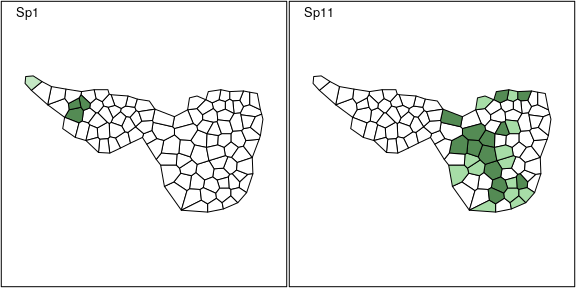## 4.2 Moran’s coefficient of spatial autocorrelation The element mafragh$nb contains a spatial neighborhood object that can be transformed in a spatial weighting matrix as described in this section:

lw <- nb2listw(mafragh$nb) This SWM can be used to compute the level of spatial autocorrelation of a quantitative variable using the Moran’s coefficient. If we consider the $$n \times 1$$ vector $$\mathbf{x} = \left ( {x_1 \cdots x_n } \right )\hspace{-0.05cm}^{\top}\hspace{-0.05cm}$$ containing measurements of a quantitative variable for $$n$$ sites and $$\mathbf{W} = [w_{ij}]$$ the SWM. The usual formulation for Moran’s coefficient (MC) of spatial autocorrelation is: $MC(\mathbf{x}) = \frac{n\sum\nolimits_{\left( 2 \right)} {w_{ij} (x_i -\bar {x})(x_j -\bar {x})} }{\sum\nolimits_{\left( 2 \right)} {w_{ij} } \sum\nolimits_{i = 1}^n {(x_i -\bar {x})^2} }\mbox{ where }\sum\nolimits_{\left( 2 \right)} =\sum\limits_{i = 1}^n {\sum\limits_{j = 1}^n } \mbox{ with }i\ne j$ MC can be rewritten using matrix notation: $MC(\mathbf{x}) = \frac{n}{\mathbf{1}\hspace{-0.05cm}^{\top}\hspace{-0.05cm}\mathbf{W1}}\frac{\mathbf{z}\hspace{-0.05cm}^{\top}\hspace{-0.05cm}{\mathbf{Wz}}}{\mathbf{z}\hspace{-0.05cm}^{\top}\hspace{-0.05cm}\mathbf{z}}$ where $$\mathbf{z} = \left ( \mathbf{I}-\mathbf{1}\mathbf{1}\hspace{-0.05cm}^{\top}\hspace{-0.05cm}/n \right )\mathbf{x}$$ is the vector of centred values (i.e., $$z_i = x_i-\bar{x}$$). The function moran.mc of the spdep package allows to compute and test, by permutation, the significance of the Moran’s coefficient. A wrapper is provided by the moran.randtest function to test simultaneously and independently the spatial structure for several variables. sp.env <- SpatialPolygonsDataFrame(Sr = mafragh$Spatial, data = mafragh$env, match.ID = FALSE) maps.env <- s.Spatial(sp.env, col = fpalette(4), nclass = 4)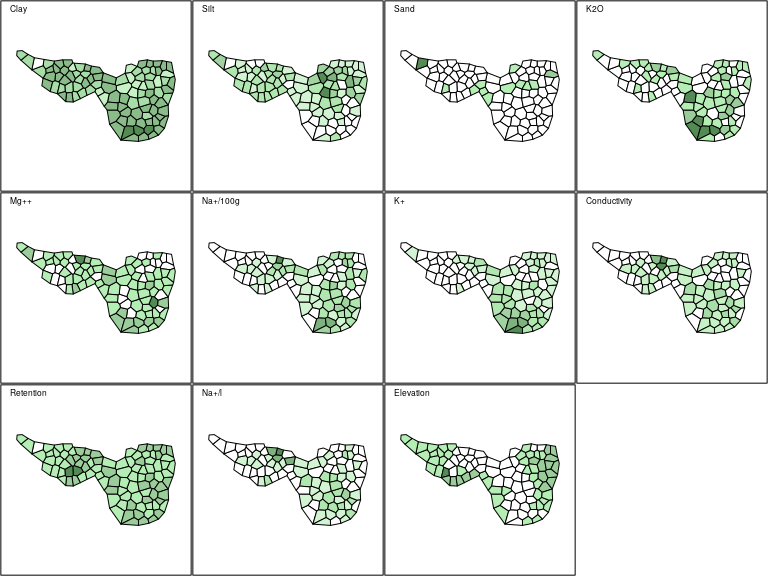MC.env <- moran.randtest(mafragh$env, lw, nrepet = 999)
MC.env
## class: krandtest lightkrandtest
## Monte-Carlo tests
## Call: moran.randtest(x = mafragh$env, listw = lw, nrepet = 999) ## ## Number of tests: 11 ## ## Adjustment method for multiple comparisons: none ## Permutation number: 999 ## Test Obs Std.Obs Alter Pvalue ## 1 Clay 0.4306240 7.211669 greater 0.001 ## 2 Silt 0.3464060 5.814863 greater 0.001 ## 3 Sand 0.1102831 2.013417 greater 0.034 ## 4 K2O 0.2913067 4.774480 greater 0.001 ## 5 Mg++ 0.1921679 3.360793 greater 0.001 ## 6 Na+/100g 0.2748070 4.572505 greater 0.001 ## 7 K+ 0.6754616 11.224141 greater 0.001 ## 8 Conductivity 0.3085226 5.177501 greater 0.001 ## 9 Retention 0.2314202 4.278060 greater 0.001 ## 10 Na+/l 0.2443979 4.276410 greater 0.001 ## 11 Elevation 0.5887046 9.825062 greater 0.001 For a given SWM, the upper and lower bounds of MC are equal to $$\lambda_{max} (n/\mathbf{1}\hspace{-0.05cm}^{\top}\hspace{-0.05cm}\mathbf{W1})$$ and $$\lambda_{min} (n/\mathbf{1}\hspace{-0.05cm}^{\top}\hspace{-0.05cm}\mathbf{W1})$$ where $$\lambda_{max}$$ and $$\lambda_{min}$$ are the extreme eigenvalues of $$\mathbf{\Omega}$$. These extreme values are returned by the moran.bounds function: mc.bounds <- moran.bounds(lw) mc.bounds ## Imin Imax ## -0.5732037 1.0028499 Hence, it is possible to display Moran’s coefficients computed on environmental variables and the minimum and maximum values for the given SWM: env.maps <- s1d.barchart(MC.env$obs, labels = MC.env\$names, plot = FALSE, ylim = 1.1 * mc.bounds, p1d.hori = FALSE)
addline(env.maps, h = mc.bounds, plot = TRUE, pline.col = 'red', pline.lty = 3)